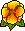## Computation of resonance frequencies for Maxwell equations in non smooth domains

Martin Costabel, Monique DaugeWe address the computation by finite elements of the non-zero eigenvalues of the (curl, curl) bilinear form with perfect conductor boundary conditions in a polyhedral cavity. One encounters two main difficulties:
(i) The infinite dimensional kernel of this bilinear form (the gradient fields),
(ii) The unbounded singularities of the eigen-fields near corners and edges of the cavity.
We first list possible variational spaces with their functional properties and provide a short description of the edge and corner singularities. Then we address different formulations using a Galerkin approximation by edge elements or nodal elements.

After a presentation of edge elements, we concentrate on the functional issues connected with the use of nodal elements. In the framework of conforming methods, nodal elements are mandatory if one regularizes the bilinear form (curl, curl) in order to get rid of the gradient fields. A plain regularization with the (div, div) bilinear form converges to a wrong solution if the domain has reentrant edges or corners. But remedies do exist. We will present the method of addition of singular functions, and the method of regularization with weight, where the (div, div) bilinear form is modified by the introduction of a weight which can be taken as the distance to reentrant edges or corners.Survey paper, in connection to the LMS Durham Symposium
Computational methods for wave propagation in direct scattering. 15-25 July 2002, Durham, UK

Published in the Lecture notes in computational science and engineering Vol. 31, Springer (2003).PDF file (388 k)July 20, 2002. Slides of the talk by M.D. given at the LMS Durham SymposiumPDF file (168 k)

July 20, 2002. Slides of the talk by M.C. given at the LMS Durham Symposium
(More about the weighted regularization -- including numerical experiments in 2D and 3D)Talk given by M.C. in Durham.